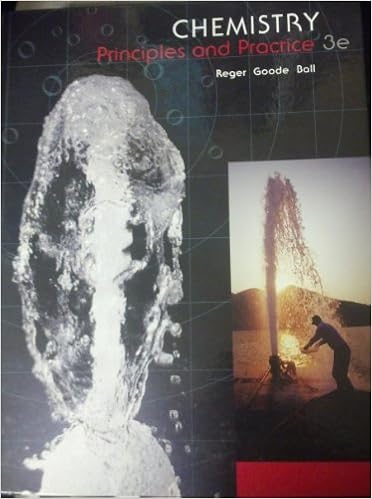If k c1 is the equilibrium constant for the reaction

• Notes
• BailiffBookPorcupine4552
• 25
• 100% (1) 1 out of 1 people found this document helpful

This preview shows page 12 - 14 out of 25 pages.

We have textbook solutions for you!
The document you are viewing contains questions related to this textbook.The document you are viewing contains questions related to this textbook.
Chapter 11 / Exercise 11.19
Chemistry: Principles and Practice
Reger ChemExpert Verified
2) If K C1 is the equilibrium constant for the reaction: H 2 SO 4 (aq) + H 2 O(l) H 3 O + (aq) + HSO 4 (aq) and K C2 is the equilibrium constant for the reaction: HSO 4 (aq) + H 2 O(l) H 3 O + (aq) + SO 4 2 (aq) what is the equilibrium constant for the overall equilibrium: H 2 SO 4 (aq) + 2 H 2 O(l) 2 H 3 O + (aq) + SO 4 2 (aq) a) K C2 (the slower step) b) K C1 + K C2 c) K C1 /K C2 d) K C1 – K C2 e) K C1 x K C2
3) If K c = 7.04 x 10 -2 for the reaction 2 HBr(g) H 2 (g) + Br 2 (g), what is the value of K c for the reaction: ½ H 2 (g) + ½ Br 2 (g) HBr(g)?
4) If K c is the equilibrium constant for a forward reaction, what is K c for two times the reverse reaction?
5) A reaction reaches dynamic equilibrium at a given temperature when .
6) What is K p at 1273 ° C for the reaction 2 CO (g) + O 2 (g) 2 CO 2 (g) if K c is 2.24 x 10 22 at the same temperature? a) 1.35 b) 5.66 x 10 -21 c) 1.77 x 10 20 d) 5.66 x 10 21
7) At a certain temperature bromine and nitric oxide react to form nitrosyl bromide: Br 2 (g) + NO (g) 2 NOBr (g) When initial amounts of Br 2 , NO, and NOBr are mixed, the concentration of NOBr increases. Which statement below is true about the initial mixture? c c c .
- 12 -
We have textbook solutions for you!
The document you are viewing contains questions related to this textbook.The document you are viewing contains questions related to this textbook.
Chapter 11 / Exercise 11.19
Chemistry: Principles and Practice
Reger ChemExpert Verified
1011ExamPrepWorksheet.doc 8) The equilibrium constant K p equals 3.40 at 25 ° C for the isomerization reaction cis -2-butene ' trans -2-butene If a flask initially contains 1.00 atm of each gas, in what direction will the reaction proceed to reach equilibrium?
9) Which statement about the change of a concentration of a solid during a reaction is most correct?
10) Once the following equation has been correctly balanced, determine which of the following equilibrium constant expressions for K c best applies. C (s) + H 2 O (g) ' C 2 H 5 OH (g) + O 2 (g) (not balanced) a) [ ] [ [ ] [ ] ] O H C O OH H C 2 2 5 2 c = b) [ ] [ ] [ ] [ ] 3 2 2 2 5 2 c O H C O OH H C K = c) [ ] [ [ ] ] O H O OH H C 2 2 5 2 c = d) [ ] [ ] [ ] 3 2 2 5 2 c O H O OH H C K = K K
•••